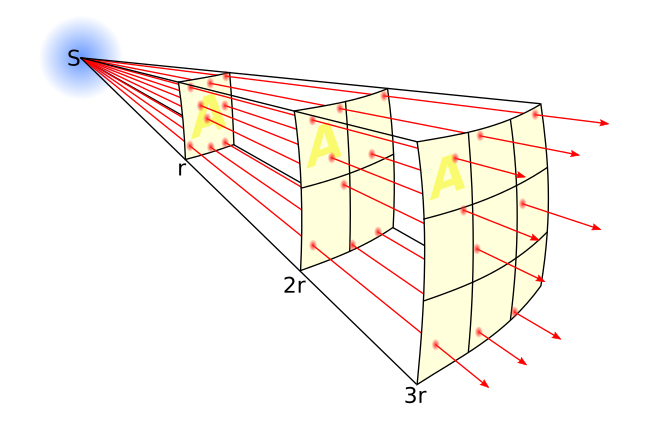4.9

## UNSW SydneyThe flux generated by a point gravitational force

# Gravitation and Newton's inverse square law

Isaac Newton was a giant of modern science, and one of the key architects of the modern world. One of his major insights was that the force of gravitational attraction between two bodies had a precise mathematical aspect.

In this step we look at Newton’s inverse square law: an important example of a power law that falls outside of the inverse relations we have talked about previously, but is still closely related to it.

## Inverse square laws

A variables $\normalsize{y}$ is related to a variable $\normalsize{x}$ by an inverse square law if we can write $\normalsize{y=a/x^2}$ for some constant $\normalsize{a}$. Here is the case when $\normalsize{a=1}$.There are some obvious similarities with the inverse relation $\normalsize{y=1/x}$, but also some important differences. Both functions have a vertical asymptote $\normalsize{x=0}$ and a horizontal asymptote $\normalsize{y=0}$. Both pass through the point $\normalsize{[1,1]}$. However while $\normalsize{y=1/x}$ is an odd function, $\normalsize{y=1/x^2}$ is an even function.

In addition, as $\normalsize{x}$ gets large positive, $\normalsize{1/x^2}$ gets smaller much faster than $\normalsize{1/x}$. On the other hand if $\normalsize{x}$ is a small positive number approaching $\normalsize{0}$, then $\normalsize{1/x^2}$ grows much quicker than $\normalsize{1/x}$.

## Newton’s law of gravitation

In 1687 Newton published one of the most far-reaching and influential books of all time: what is now called The Principia (although the original name was longer and in Latin). In this work Newton laid the foundation for modern physics, with his elucidation of his three Laws of Motion, a statement of his Law of Gravitation, and a derivation and explanation of Kepler’s laws.Manchester John Rylands Library Isaac Newton By Paul Hermans (Own work) GFDL or CC BY-SA 3.0, via Wikimedia Commons

Newton realized that gravity is a force that exists between any two particles, no matter how big and small, and no matter how far apart they were. But the more massive they are, the larger the force, and the closer they are, the larger the force.

More precisely, the force between two masses $\normalsize{m_1}$ and $\normalsize{m_2}$ separated by a distance $\normalsize{r}$ is proportional to

It is the dependence on $\normalsize{r^2}$ in the denominator that makes this an inverse square law. The law implies that if we go twice as far from a planet, the force of gravitational attraction is only $\normalsize{1/4}$ what it was. If we go out three times as far, the force decreases to $\normalsize{1/9}$th of what it was.

## Verifying Kepler’s laws

As a remarkable application of his laws, Newton was able to deduce Kepler’s laws as consequences. In particular, having conic sections as planetary orbits, as Kepler claimed, is a mathematical consequence of the inverse square law. This was a stirring resolution to a fundamental problem that had fascinated humans for thousands of years.

It also ushered in a new era of thought and understanding about the world around us. Newton’s insights revealed that there was an order to the complexity of the world around us, and that mathematical laws ultimately lay behind a wide range of real-life phenomenon. This was to provide a major impetus to 18th century investigations into mechanics, and indeed it lies behind the remarkable growth in technology that was initiated also at this time.

It is interesting to observe that from the view of rational trigonometry, Newton’s law of gravitation shows that quadrance (square of distance) perhaps plays a more direct role physically than the distance. It turns out that in many formulas of physics, the squares of familiar quantities often play a more fundamental role than the familiar quantities themselves. This became even clearer with the work of Albert Einstein in the 20th century, when the relativistic analog of quadrance took centre stage, and mechanics went in a dramatically new direction from that laid out by Newton.

## Discussion

Newton’s derivation of Kepler’s laws from his laws of motion is such a key point in the history of thought that it ought to be more widely appreciated by young people. What do you think?